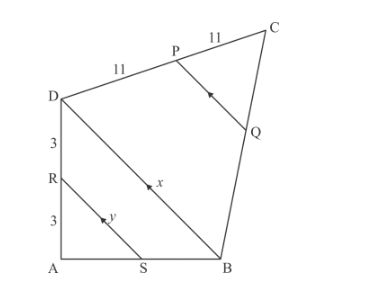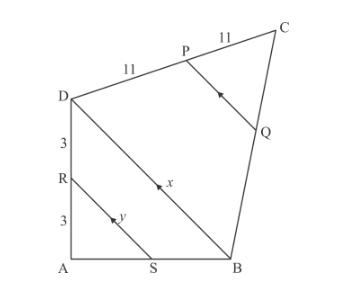# In the given figure, RS || DB || PQ. If CP = PD = 11 cm and DR = RA = 3 cm.

Question:

In the given figure, RS || DB || PQ. If CP = PD = 11 cm and DR = RA = 3 cm. Then the values of x and y are respectively.(a) 12, 10
(b) 14, 6
(c) 10, 7
(d) 16, 8

Solution:Given: RS || DB || PQ. CP = PD = 11cm and DR = RA = 3cm

To find: the value of x and y respectively.

In ∆ASR and ∆ABD,∠ASR=∠ABQ                    Corresponding angles∠A=∠A                         Common∴∆ASR ~∆ABD           AA Similarity

$\frac{\mathrm{AR}}{\mathrm{AD}}=\frac{\mathrm{AS}}{\mathrm{AB}}=\frac{\mathrm{RS}}{\mathrm{DB}}$

$\frac{3}{6}=\frac{\text { RS }}{\text { DB }}$

$\frac{1}{2}=\frac{x}{y}$

$x=2 y$

This relation is satisfied by option (d).

Hence, x = 16 cm and y = 8cm

Hence the result is $(d)$.